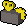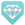For other uses, see Crystal shield (disambiguation).This article has a calculator here.Calculators determine experience and costs based on real-time prices from the Grand Exchange Market Watch.
Description?Has a 10% chance to activate on taking damage, lasting 10 seconds. 5% of damage taken per rank is totalled for this period, becoming temporary lifepoints afterwards. These last either 30 seconds or until depleted through further damage. (1 minute cooldown) (Update) Armour 3

Crystal Shield is a defensive Invention perk that, upon activation, will add a percentage of all damage taken for 10 seconds into a pool of life points. This also includes typeless damage. For the following 30 seconds, damage taken will reduce this storage of life points rather than the player's actual life points, until the separate pool is depleted or the 30 seconds have passed. It can be created in armour gizmos.

The perk has a 10% chance of activating (11% on a level 20 item), and 5% damage taken per rank is converted into the pool of life points for the duration of 10 seconds. The hit that activates the Crystal Shield will also be added to the life point pool. This perk has a 1 minute cooldown after it has activated.

If the player would die to any "hard" typeless hit (typeless damage that cannot be reduced by defensive abilities, e.g. Vorago's TeamSplit), the player will not die; they are instead left with however many life points that were stored in Crystal Shield.

When the perk triggers, it prompts the message: Your Crystal Shield perk has activated and will begin storing damage to use as ablative hitpoints. When the stored life points deplete, it prompts the message: Your crystal shield lifepoints have been depleted! When the timer ends before the stored life points deplete, it prompts the message: Your crystal shield perk has run out! When the player doesn’t receive significant damage during the perk’s activation period, it prompts the message: You did not absorb enough damage to generate a crystal shield.

Damage from poison can also trigger the perk. Any self-inflicted damage is also added to the life point pool. These include:

Average damage reduction
Rank1 0.70% (0.71%) 0.59% (0.60%) 0.67% (0.69%)
2 1.40% (1.43%) 1.18% (1.21%) 1.33% (1.38%)
3 2.09% (2.14%) 1.76% (1.81%) 2.00% (2.06%)
•is the attack speed of the enemy in game ticks.
• All numbers in parentheses refer to level 20 gear.

## Calculations

• The average ratio of damage taken,, from Crystal Shield to that of without Crystal Shield is• The average damage reduction is then, after some rearranging,Notes
• A calculator for this is at the top of this page.
• Assumptions :
• The pool of life points is always depleted.
• The enemy is always attacking at the same attack speed.
•is the proc chance of Crystal Shield to activate (.10 normally, .11 if the Crystal Shield perk is on level 20 gear).
•whenor. This represents summing over all possibilities where integerrepresents the amount of hits prior to the proc of Crystal Shield. The probability of Crystal Shield proccing on hitis therefore.
•is the rank of the Crystal Shield perk.
•is the time of the absorption phase of Crystal Shield. This is taken to be 16 game ticks.
•is the cooldown time of Crystal Shield. This is taken to be 100 game ticks.
•is the attack speed of the enemy in game ticks.
•is therandom value uniformly sampled between the enemy's minimum hit and maximum hit. Thedescribes different sets of hits. The sets are regenerated for every new value of.
•haselements with integer. This is the damage taken before the perk procs.
• Clarification : This does mean that forthat there are no elements in this set.
•haselements with integer. This is the damage taken during the absorption phase.
•haselements with integer. This is the damage taken from the end of the absorption phase until the chance for Crystal Shield to proc is no longer on cooldown.
• The set of values infor integeris the same in both the numerator and denominator of the ratios for any given.
• The flooring functionis used in theterm because the game truncates the amount to be added into the pool. This is important because the amount added to the pool is directly related to the amount of damage taken per hit during the absorption phase. This means that for, if any damage taken is under 20 during the absorption phase, it will not contribute to the shield. Similarly, forand, any damage taken that is under 10 and 7, respectively, during the absorption phase will not contribute to the shield. If each individual hit during the absorption phase is under these respective lower limit values per Crystal Shield rank, then a shield will not form.

Simplifications
• This can be simplified if the assumption is that every value ofinteger, integer, integer, is taken to be the same. In this scenario, there is no random element and the aboveis then only dependent on the proc chance and the attack speed of the enemy. This simplification is increasingly accurate in the limit of large. A value of constant damage taken under 80 will have a noticeable effect. Three examples are provided (,, and) at the top of the page. All examples are using large enough values ofsuch that the values should not change to 2 decimal places in percent.

Using these simplifications, the damage reduction reduces toProof of the simplification
Regarding Crystal Shield

We start from the main equation:The second summation within the brackets in de numerator can be split up.Split up the damage reduction component.The first term of the numerator is equal to the denominator.If we want to know the damage reduction over a large number of hits, we could use the statistical average of the received damage, this meansbecomes a constant value. We also drop the floor function in the numerator and add a valueto correct for the error.The second summation in the numerator has no terms that change on increasing, the summation can thus be simplified to a product of the range of the index and the summands.Place terms not dependent onoutside of the summations.Drop common term in numerator and denominator.

$\displaystyle r_{avg} = 1 - \frac{ \left\lceil{\frac{t_{abs}}{t_{AS}}}\right\rceil \left(.05 \times R - \frac{\phi}{\bar{d}}\right) \sum\limits_{n=0}^{\infty} \left(1-p\right)^{n} }{ \sum\limits_{n=0}^{\infty} \left(1-p\right)^{n} \left[ n + \left\lceil{\frac{t_{abs}}{t_{AS}}}\right\rceil + \left\lceil{\frac{t_{cd}-t_{abs}}{t_{AS}}}\right\rceil \right] } \tag{1}$

To simplify even further we need to find the infinite sum equivalents of the remaining summations. Since(p = perk proc chance), the following is true[math 1]

$\displaystyle \tag{2} \sum\limits_{n=0}^{\infty} ax^{n} = \frac{a}{1-x}$

Take the derivative of (2):

\displaystyle \begin{aligned} \sum\limits_{n=0}^{\infty} anx^{n-1} &= \frac{a}{(1-x)^2} \\ \sum\limits_{n=0}^{\infty} a(n+1)x^{n} &= \frac{a}{(1-x)^2} \\ \sum\limits_{n=0}^{\infty} anx^{n} + \sum\limits_{n=0}^{\infty} ax^{n} &= \frac{a}{(1-x)^2} \tag{3}\end{aligned}

The second term in (3) is equal to (2):Combine (2) and (3) with diffent constants:

\displaystyle \begin{aligned} \sum\limits_{n=0}^{\infty} anx^{n} + \sum\limits_{n=0}^{\infty} bx^{n} &= \frac{ax}{(1-x)^2} + \frac{b}{1-x}\\ \sum\limits_{n=0}^{\infty} x^{n}(an+b) &= \frac{ax + b(1-x)}{(1-x)^2} \tag{4}\end{aligned}

If we takeandin (2) we get:

$\displaystyle \sum\limits_{n=0}^{\infty} (1-p)^{n} = \frac{1}{p} \tag{5}$

For,andin (4), we get:

$\displaystyle \sum\limits_{n=0}^{\infty} (1-p)^{n} \left[ n + \left\lceil{\frac{t_{abs}}{t_{AS}}}\right\rceil + \left\lceil{\frac{t_{cd}-t_{abs}}{t_{AS}}}\right\rceil \right] = \frac{(1-p) + \left(\left\lceil{\frac{t_{abs}}{t_{AS}}}\right\rceil + \left\lceil{\frac{t_{cd}-t_{abs}}{t_{AS}}}\right\rceil\right)p}{p^2} \tag{6}$

The summations (5) and (6) occur in (1) and can be substituted:Where. This represents the rounding error introduced by dropping the floor function., so forwe have <4% error for ignoringand can therefor safely be ignored for most practical situations.

## Sources

MaterialRarityPerk ranks with X materials
12345
Clear partsCommon01111
Subtle componentsUncommon111–21–31–3
Faceted componentsRare1112–33